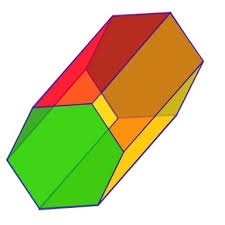# Hexagonal 29141

Calculate the volume and surface of a regular hexagonal prism with a base edge a = 30 m and a side edge b = 50 m.

V =  116913.4295 m3
S =  13676.5372 m2

### Step-by-step explanation:Did you find an error or inaccuracy? Feel free to write us. Thank you!

Tips for related online calculators
Need help calculating sum, simplifying, or multiplying fractions? Try our fraction calculator.